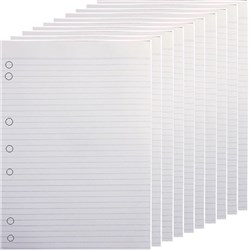Select Office Supplies

\$1.80 \$1.99 inc GST ex GST (EACH)
Increase value Decrease value
\$1.18 \$1.30 inc GST ex GST (EACH)
Increase value Decrease value
\$2.71 \$2.99 inc GST ex GST (EACH)
Increase value Decrease value
\$2.71 \$2.99 inc GST ex GST (EACH)
Increase value Decrease value
\$3.62 \$3.99 inc GST ex GST (EACH)
Increase value Decrease value
\$2.08 \$2.29 inc GST ex GST (EACH)
Increase value Decrease value
\$1.08 \$1.19 inc GST ex GST (EACH)
Increase value Decrease value
\$2.95 \$3.25 inc GST ex GST (EACH)
Increase value Decrease value
\$4.77 \$5.25 inc GST ex GST (EACH)
Increase value Decrease value
\$1.80 \$1.99 inc GST ex GST (EACH)
Increase value Decrease value
\$2.71 \$2.99 inc GST ex GST (EACH)
Increase value Decrease value
\$1.80 \$1.99 inc GST ex GST (EACH)
Increase value Decrease value
\$22.72 \$24.99 inc GST ex GST (PACK)
Increase value Decrease value
\$1.71 \$1.89 inc GST ex GST (EACH)
Increase value Decrease value
\$2.71 \$2.99 inc GST ex GST (EACH)
Increase value Decrease value
\$0.99 \$1.09 inc GST ex GST (EACH)
Increase value Decrease value
\$21.80 \$23.99 inc GST ex GST (PACK)
Increase value Decrease value
\$4.53 \$4.99 inc GST ex GST (EACH)
Increase value Decrease value
\$3.62 \$3.99 inc GST ex GST (EACH)
Increase value Decrease value
\$3.62 \$3.99 inc GST ex GST (EACH)
Increase value Decrease value
\$29.99 \$32.99 inc GST ex GST (PACK)
Increase value Decrease value
\$17.26 \$18.99 inc GST ex GST (PACK)
Increase value Decrease value
\$9.99 \$10.99 inc GST ex GST (PACK)
Increase value Decrease value
\$2.95 \$3.25 inc GST ex GST (EACH)
Increase value Decrease value\$7.27 \$8.00 inc GST ex GST (EACH)
Increase value Decrease value
\$53.63 \$59.00 inc GST ex GST (EACH)
Increase value Decrease value
\$25.00 \$27.50 inc GST ex GST (EACH)
Increase value Decrease value
\$42.72 \$47.00 inc GST ex GST (EACH)
Increase value Decrease value
##### Products to compare:
Comparing Products